# Part 2: Evaluating Foundation Models (CLIP) using Encord Active

August 22, 2023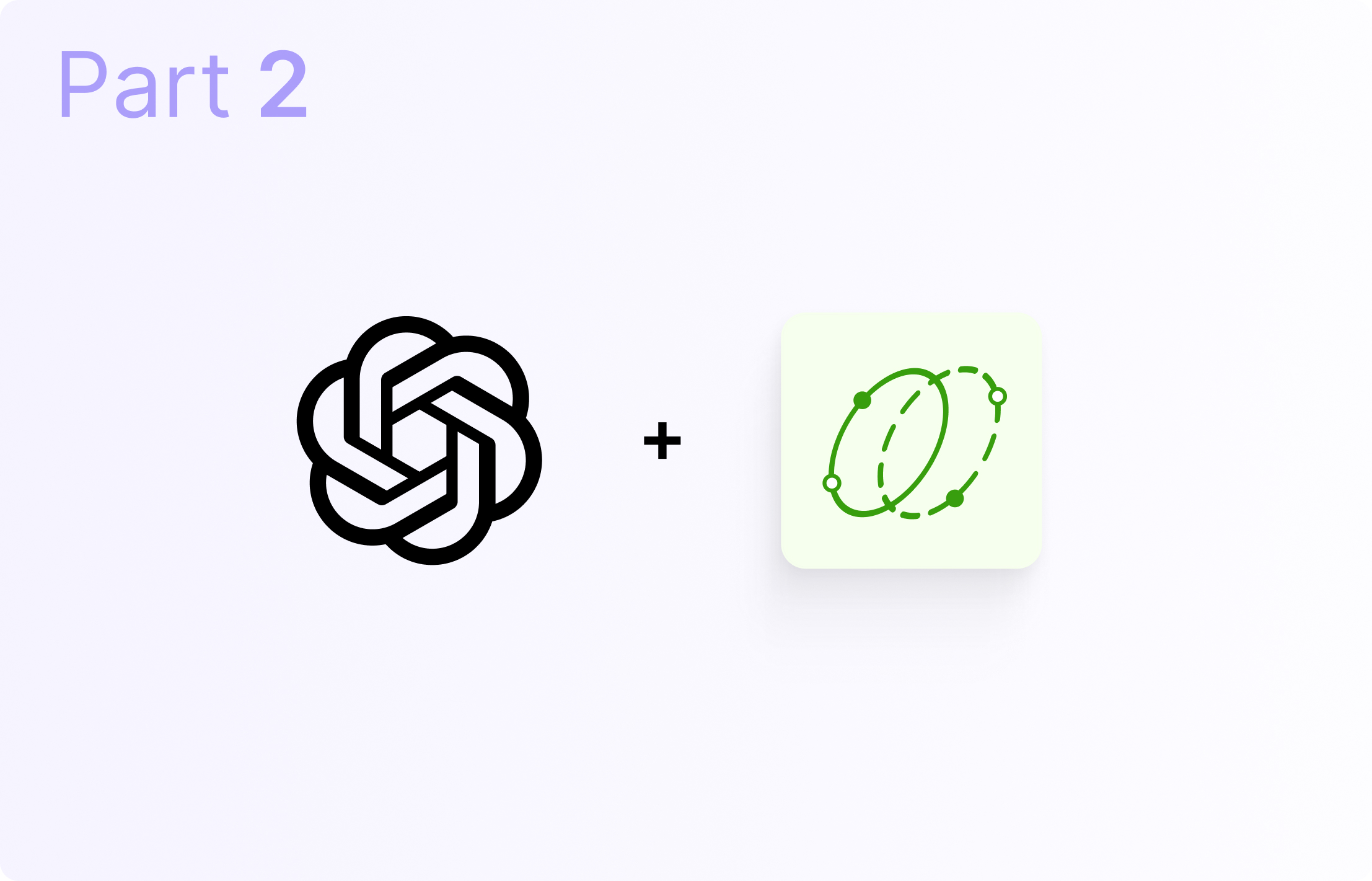In the first article of this series on evaluating foundation models using Encord Active, you applied a CLIP model to a dataset that contains images of different facial expressions. You also saw how you could generate the classifications for the facial expressions using the CLIP model and import the predictions into Encord Active.

To round up that installment, you saw how Encord Active can help you evaluate your model quality by providing a handy toolbox to home in on how your model performs on different subsets of data and metrics (such as image singularity, redness, brightness, blurriness, and so on).

In this installment, you will focus on training a CNN model on the ground truth labels generated by the CLIP model. Toward the end of the article, you will import the dataset, ground truth labels, and model into Encord Active to evaluate the model and interpret the results to analyze the quality of your model.

Let’s jump right in! 🚀

## Train CNN Model on A Dataset with Ground Truth Labels

In this section, you will train a CNN on the dataset created from labels predicted by the CLIP model. We saved the name of the dataset folder as Clip_GT_labels in the root directory—the code snippet for creating the new dataset from the CLIP predictions.Remember to check out the complete code for the article in this repository.

Create a new Python script named “train_cnn.py” in the root directory. Import the required libraries:

import torch
import torch.nn as nn
import torch.optim as optim
import torchvision.transforms as transforms
import torchvision.datasets as datasets
from tqdm import tqdm

Next, define transforms for data augmentation and load the dataset:

# Define the data transformations
train_transforms = transforms.Compose([
transforms.Resize((256, 256)),
transforms.RandomHorizontalFlip(),
transforms.RandomVerticalFlip(),
transforms.ToTensor(),
])

val_transforms = transforms.Compose([
transforms.Resize((256, 256)),
transforms.ToTensor(),
])

train_dataset = datasets.ImageFolder(
r'Clip_GT_labels\Train',
transform=train_transforms
)

val_dataset = datasets.ImageFolder(
r'Clip_GT_labels\Val',
transform=val_transforms
)

val_loader = DataLoader(val_dataset, batch_size=32, shuffle=False)

Next, define the CNN architecture, initialize the model, and define the loss function and optimizer:

# Define the CNN architecture
class CNN(nn.Module):
def __init__(self, num_classes=7):
super(CNN, self).__init__()
# input shape (3, 256, 256)
self.conv1 = nn.Conv2d(3, 16, kernel_size=3, padding=1)
self.relu1 = nn.ReLU(inplace=True)
self.pool1 = nn.MaxPool2d(kernel_size=2)
# shape (16, 128, 128)

# input shape (16, 128, 128)
self.conv2 = nn.Conv2d(16, 32, kernel_size=3, padding=1)
self.relu2 = nn.ReLU(inplace=True)
self.pool2 = nn.MaxPool2d(kernel_size=2)
# output shape (32, 64, 64)

# input shape (32, 64, 64)
self.conv3 = nn.Conv2d(32, 64, kernel_size=3, padding=1)
self.relu3 = nn.ReLU(inplace=True)
self.pool3 = nn.MaxPool2d(kernel_size=2)
# output shape (64, 32, 32)

# input shape (64, 32, 32)
self.conv4 = nn.Conv2d(64, 32, kernel_size=3, padding=1)
self.relu4 = nn.ReLU(inplace=True)
self.pool4 = nn.MaxPool2d(kernel_size=2)
# output shape (32, 16, 16)

self.fc1 = nn.Linear(32 * 16 * 16, 128)
self.relu5 = nn.ReLU(inplace=True)
self.dropout = nn.Dropout(0.5)
self.fc2 = nn.Linear(128, num_classes)

def forward(self, x):
x = self.conv1(x)
x = self.relu1(x)
x = self.pool1(x)
x = self.conv2(x)
x = self.relu2(x)
x = self.pool2(x)
x = self.conv3(x)
x = self.relu3(x)
x = self.pool3(x)
x = self.conv4(x)
x = self.relu4(x)
x = self.pool4(x)
x = x.view(-1, 32 * 16 * 16)
x = self.fc1(x)
x = self.relu5(x)
x = self.dropout(x)
x = self.fc2(x)
return x

# Initialize the model and define the loss function and optimizer
model = CNN()
criterion = nn.CrossEntropyLoss()
optimizer = optim.Adam(model.parameters(), lr=0.001)

Finally, here’s the code to train the CNN on the dataset and export the model:

# Train the model
device = torch.device('cuda' if torch.cuda.is_available() else 'cpu')
model.to(device)

num_epochs = 50
best_acc = 0.0

for epoch in range(num_epochs):
train_loss = 0.0
train_acc = 0.0

model.train()
images = Variable(images.to(device))
labels = Variable(labels.to(device))

outputs = model(images)
loss = criterion(outputs, labels)
loss.backward()
optimizer.step()

train_loss += loss.item() * images.size(0)
_, preds = torch.max(outputs, 1)
train_acc += torch.sum(preds == labels.data)

train_loss = train_loss / len(train_dataset)
train_acc = train_acc / len(train_dataset)

val_loss = 0.0
val_acc = 0.0

model.eval()
images = images.to(device)
labels = labels.to(device)

outputs = model(images)
loss = criterion(outputs, labels)

val_loss += loss.item() * images.size(0)
_, preds = torch.max(outputs, 1)
val_acc += torch.sum(preds == labels.data)

val_loss = val_loss / len(val_dataset)
val_acc = val_acc / len(val_dataset)

print('Epoch [{}/{}], Train Loss: {:.4f}, Train Acc: {:.4f}, Val Loss: {:.4f}, Val Acc: {:.4f}'.format(epoch+1, num_epochs, train_loss, train_acc, val_loss, val_acc))

if val_acc > best_acc:
best_acc = val_acc
torch.save(model.state_dict(), 'cnn_model.pth')

Now, execute the script:

# Go back to root folder
cd ..

# execute script
python train_cnn.py

If the script executes successfully, you should see the exported model in your root directory:

├── Clip_GT_labels
├── EAemotions
├── classification_transformer.py
├── cnn_model.pth
├── emotions
├── make_clip_predictions.py
└── train_cnn.py

## Evaluate CNN Model Using Encord Active

In this section, you will perform the following task:

• Create a new Encord project using the test set in the Clip_GT_labels dataset.
• Load the trained CNN model above (“cnn_model.pth”) and use it to make predictions on the test.
• Import the predictions into Encord for evaluation.

### Create An Encord Project

Just as you initially created a project in the first article, use the test set in the Clip_GT_labels dataset to initialize a new Encord project. The name specified here for the new project is EAsota.

# Create project
encord-active init --name EAsota --transformer classification_transformer.py Clip_GT_labels\Test

# Change to project directory
cd EAsota

# Store ontology
encord-active print --json ontology

### Make Predictions using CNN Model

In the root directory, create a Python script with the name cnn_prediction.py.

Load the new project into the script:

# Import encord project
project_path = r'EASOTA'

)

)

Next, instantiate the CNN model and load the artifact (saved state):

# Create an instance of the model
model = CNN()

# Load the saved state dictionary file
model_path = 'cnn_model.pth'
model.load_state_dict(torch.load(model_path))

Using the same procedures as in the previous article, make predictions on the test images and export the predictions by appending them to the predictions_to_import list:

model.eval()
output = model(image_transformed.to(device).unsqueeze(dim=0))
class_id = output.argmax(dim=1, keepdim=True).item()
model_prediction = project_ontology['classifications'][classes[class_id]]
my_predictions.append(classes[class_id])
confidence = output.softmax(1).tolist()[class_id]

If you included the same custom metrics, you should have an output in your console: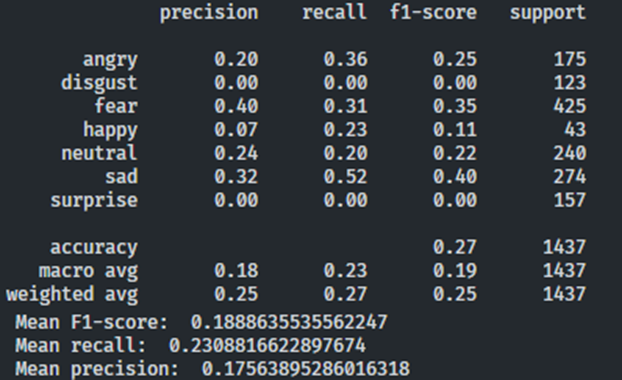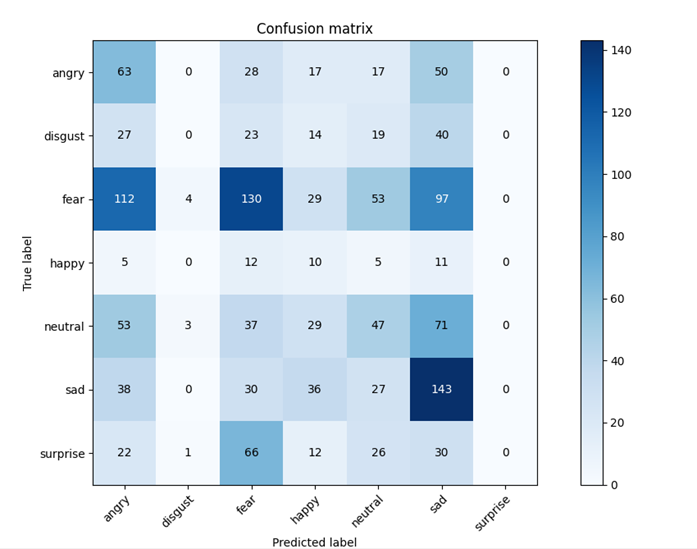### Import Predictions into Encord

In the EAsota project, you should find the predictions.pkl file, which stores the predictions from the CNN model.

Import the predictions into Encord Active for evaluation:

# Change to Project directory
cd ./EAsota

# Import Predictions
encord-active import predictions predictions.pkl

# Start encord-active webapp server
encord-active visualize

Below is Encord Active’s evaluation of the CNN model’s performance: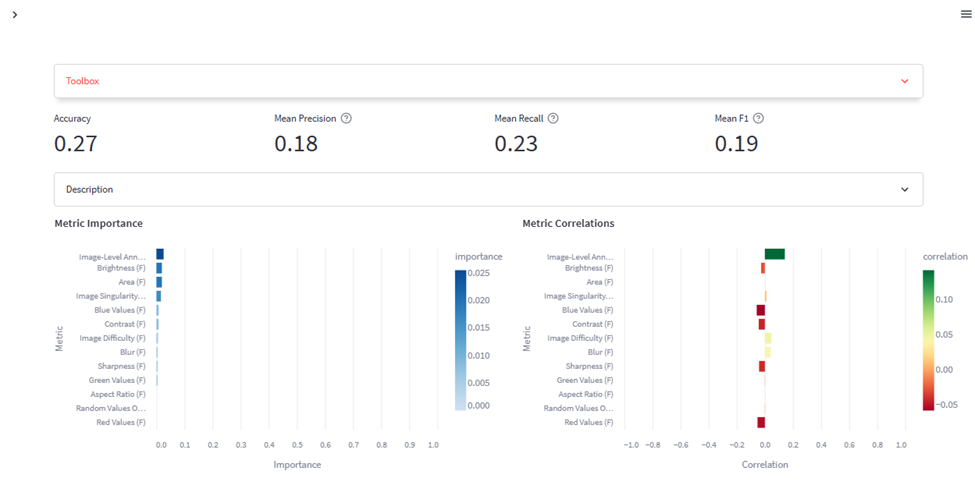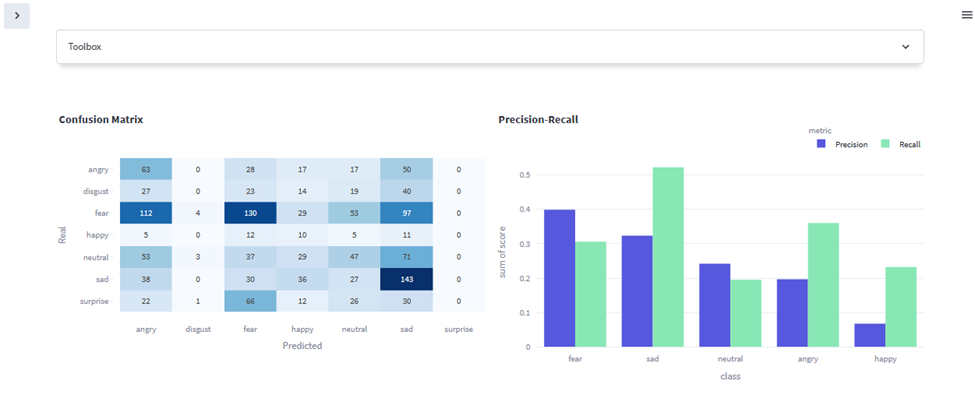### Interpreting the model's results

The classification metrics provided show that the model is performing poorly. The accuracy of 0.27 means that only 27% of the predictions are correct. The mean precision of 0.18 indicates that only 18% of the positive predictions are correct, and the mean recall of 0.23 means that only 23% of the instances belonging to a class are captured.

The mean F1 score of 0.19 reflects the overall balance between precision and recall, but it is still low. These metrics suggest that the model is not making accurate predictions and needs significant improvement.

Encord also visualized each metric's relative importance and correlation to the model's performance. For example, increasing the image-level annotation quality (P), slightly reducing the brightness of the images in the dataset, etc., can positively impact the model’s performance.

## What have you learned in this series?

Over the past two articles, you have seen how to use a CLIP model and train a CNN model for image classification.

Most importantly, you learned to use Encord Active, an open-source computer vision toolkit, to evaluate the model’s performance using an interactive user interface. You could also visually get the accuracy, precision, f1-score, recall, confusion matrix, feature importance, etc., from Encord Aactive.

Check out the Encord Active documentation to explore other functionalities of the open-source framework for computer vision model testing, evaluation, and validation. Check out the project on GitHub, leave a star 🌟 if you like it, or leave an issue if you find something is missing—we love feedback!Stephen Oladele is a Developer Advocate and an MLOps Technical Content Creator at Encord. He has significant experience building and managing data communities, and you will find him learning and discussing machine learning topics across Discord, Slack and Twitter. Stephen has a background... see more#### Discuss this blog on Slack

Join the Encord Developers community to discuss the latest in computer vision, machine learning, and data-centric AI

Join the community
###### Subscribe